Courses

# Load-Flow Study of Power Systems

## 10 Questions MCQ Test Topicwise Question Bank for Electrical Engineering | Load-Flow Study of Power Systems

Description
This mock test of Load-Flow Study of Power Systems for Electrical Engineering (EE) helps you for every Electrical Engineering (EE) entrance exam. This contains 10 Multiple Choice Questions for Electrical Engineering (EE) Load-Flow Study of Power Systems (mcq) to study with solutions a complete question bank. The solved questions answers in this Load-Flow Study of Power Systems quiz give you a good mix of easy questions and tough questions. Electrical Engineering (EE) students definitely take this Load-Flow Study of Power Systems exercise for a better result in the exam. You can find other Load-Flow Study of Power Systems extra questions, long questions & short questions for Electrical Engineering (EE) on EduRev as well by searching above.
QUESTION: 1

### Assumption of decoupling is valid only if

Solution:

Assumption of decoupling is valid in load flow study only if resistance of the line is negligible in comparison to the reactance of the line.

QUESTION: 2

### In a load flow solution Vi = 1.083∠150 pu and Vk = 0.986∠-20 pu. What is the direction of active power (P) and reactive power (Q) flow in the line i - k?

Solution:

Since active power flows from leading bus to lagging bus therefore, P will flow from i to k.
Since, reactive power flows from high potential bus to low potential bus therefore, Q will flow from i to k.

QUESTION: 3

### The slack bus has to be a

Solution:

Out of the 15% of Generator buses in a power system, one bus is taken as a slack bus which take care of losses occuring in the system. At a generator bus P and V are known. Hence, a slack bus can be a PV bus.

QUESTION: 4

Providing synchronous condensers at a load bus will control

Solution:

A synchronous condeser regulates Q and thus controls power factor of the system.

QUESTION: 5

Generally in load flow solutions the load is modelled as a

Solution:
QUESTION: 6

At a slack bus

Solution: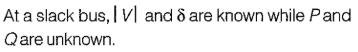QUESTION: 7

The complex power injections at a bus are as shown below. Two lines are connected drawing powers as shown in figure below. Sx is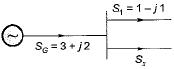Solution: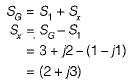QUESTION: 8

When the bus voltage magnitudes at the two ends of a transmission line are equal, if the line is lossless, then Sij is related to Sji by which of the following relationships? (Sij is complex power from bus i to j)

Solution:
QUESTION: 9

If S = VI*, P is positive and Q is negative, the network is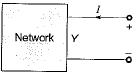Solution:

Given, S = ( P - j Q )... for given network
Since P is positive, therefore the given network absorbs P.
Since Q is negative, therefore the given network absorbs -Q or delviers +Q.

QUESTION: 10

In a power system with 100 buses, 1 bus is taken as slack bus, 15 buses are P\/ buses and rest are PQ buses. The number of variables to be solved by NR method in polar coordinates is

Solution: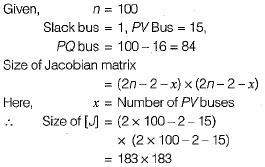Hence, number of variables to be solved by NR method in polar coordinates = 183.